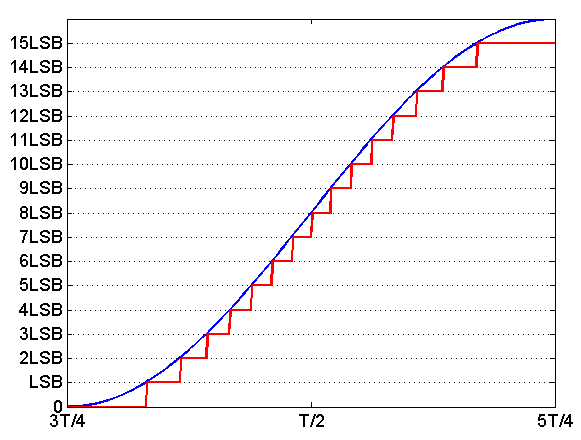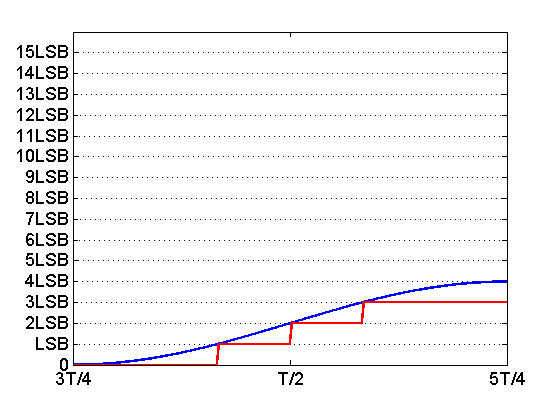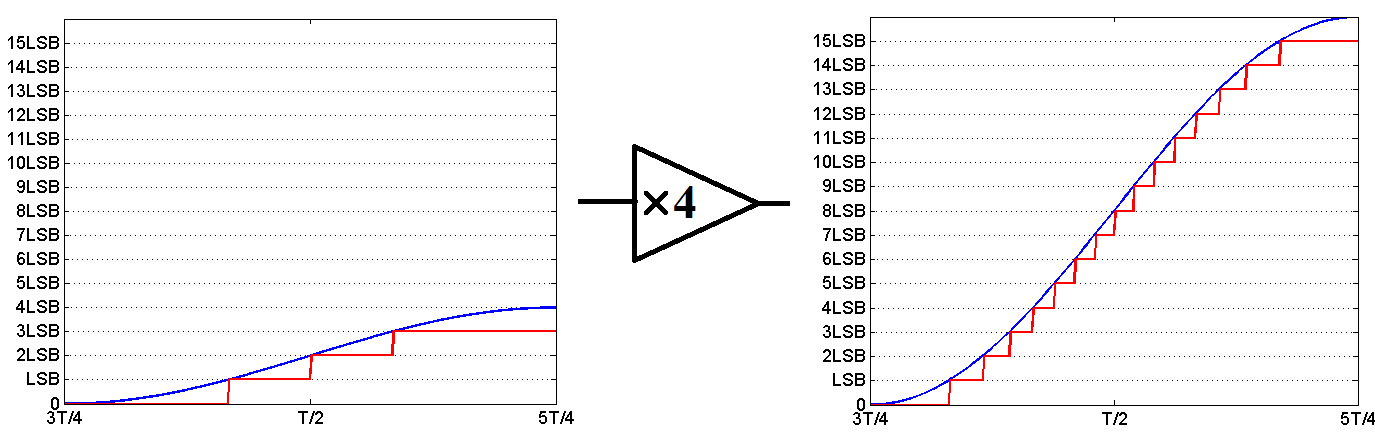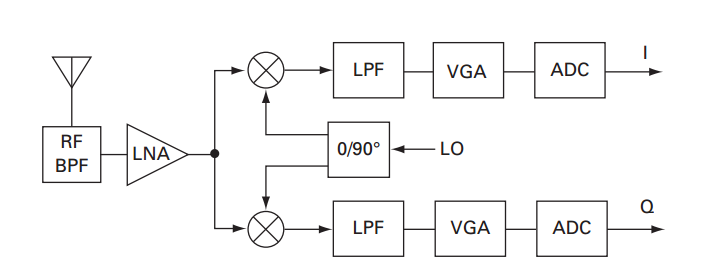Technical Article

# Understanding the Dynamic Range Specification of an ADC

June 11, 2018 by Dr. Steve Arar

Dynamic Range (DR) is a common performance metric for analog-to-digital converters (ADCs). This specification becomes particularly important in applications such as wireless communications and instrumentation. This article will review the definition of dynamic range. Then, we’ll see that to efficiently utilize the dynamic range of an ADC, the amplitude of the input signal should be adjusted to the reference voltage of the ADC.

The dynamic range is defined as the ratio between the largest and smallest values that the ADC can reliably measure. For an ADC, the dynamic range is related to the number of bits that are used to digitize the analog signal. Consider an ideal N-bit ADC. The minimum value that can be detected is one least significant bit (LSB). The maximum value is $$2^N-1$$ times the LSB value. Hence, in terms of decibels, the dynamic range of the ADC will be

$$DR = 20 \ log_{10} \Big ( \frac{(2^N-1)LSB}{LSB} \Big ) \approx 6.02 \times N \ \; (dB)$$

Therefore, with a 10-bit ADC, we would expect a dynamic range of 60.2 dB. This means that the ADC would be able to resolve signal amplitudes from x to about 1000x where x is the minimum that can be detected.

### Why the Dynamic Range Is Important: An Example

The dynamic range specification can be particularly useful in communication applications where the strength of the received signal can vary dramatically. Note that a desired radio should reliably receive both very weak and very strong signals. This large dynamic range of the receiver is significantly affected by the dynamic range of the ADC utilized in the receive chain.

The ADC has a particular dynamic range and we generally adjust the amplitude of the received signal to efficiently utilize the available dynamic range of the ADC. This will be further clarified in the next section.

Assume that we have an ideal four-bit ADC with a reference voltage of 2 volts. In this case, the ADC will use 16 discrete levels to quantize the input signal. Assume that we apply $$x_1(t)=1^{volt}+1^{volt} sin(2 \pi f_1t)$$ to this ADC where $$f_1$$ is the frequency of the sine. The ADC will compare the amplitude of the input signal with its 16 discrete levels.

Based on this comparison, the digital representation of the sine will be generated. For example, the process of comparing the input signal with the 16 discrete levels of the ADC may lead to the red curve shown in Figure 1. In this figure, $$T$$ is equal to $$\tfrac{1}{f_1}$$. Now, a digital code will be used to represent each level of the obtained staircase approximation.

For example, when the value of the red curve is equal to 4 times the LSB, the output of our four-bit ADC will be 0100.##### Figure 1. The analog signal applied to the ADC (blue) and its staircase equivalent (red).

Now, assume that we use the same ADC to digitize $$x_2(t)=0.25^{volt}+0.25^{volt}sin(2 \pi f_1t)$$. The staircase approximation of this signal will be as shown in Figure 2.##### Figure 2

Note that since the reference voltage of the ADC has not changed, its LSB is still $$\tfrac{2}{16}$$ volt. As shown in Figure 2, the maximum of the input signal, which is 4LSB, is considerably smaller than the reference voltage of the ADC. You may say that Figure 2 shows the expected functionality for our ADC and there is nothing wrong with it.

To have a better insight, assume that this ADC is utilized in a hypothetical receiver. During the normal operation of the receiver, the ADC should digitize $$x_1(t)$$ which was depicted in Figure 1. That’s why the reference voltage of the ADC is chosen to be equal to the maximum value of the input analog signal which is 2 volts. However, assume that, in certain geographic locations, the received signal is weak and the ADC must digitize $$x_2(t)$$ as illustrated in Figure 2. For the latter case, we are using only four discrete levels to digitize the analog signal.

In Figure 1, it’s almost easy to see that the red curve is a staircase approximation of $$x_1(t)$$. However, in Figure 2, we can hardly recognize that the red curve approximates a part of a sine waveform. This is due to the fact that the second experiment employs a smaller number of levels to approximate the analog signal. In this case, the levels greater than or equal to 4LSB are not utilized at all. And the ADC is equivalent to a two-bit ADC with a reference voltage of 4LSB. Effectively, the number of bits of the ADC is reduced from four in Figure 1 to two in Figure 2.

To circumvent this reduction in the effective number of bits of the ADC, we can simply multiply the weak signal by a factor of four and adjust its maximum to the reference voltage of the ADC. Then, if necessary, in the following stages of the system, we can take into account that an extra multiplication by four was applied to the input. This is shown in Figure 3.##### Figure 3. Click to enlarge.

In general, when the input signal has a wide dynamic range, we can employ a low-noise variable gain amplifier (VGA) before the ADC. For example, as shown in Figure 4, a receiver may employ a VGA before analog-to-digital conversion. The gain of the VGA will be dynamically adjusted based on the amplitude of the input signal. This will effectively extend the dynamic range of the cascade of the VGA and ADC.##### Figure 4

The Analog Devices Circuit Note presents an example for putting a VGA before the ADC. Without the VGA, the 10-bit ADC provides a dynamic range of about 60 dB. However, by employing a low-noise VGA that has a gain range of 48 dB, the cascade of the VGA and the 10-bit ADC offers a dynamic range greater than 100 dB.

### Conclusion

Certain applications, such as wireless communications, require a large dynamic range for the system. Usually, the dynamic range of the employed ADC can limit the overall dynamic range of the system. In such cases, we may have to put a low-noise VGA before the ADC. The VGA will amplify weak signals and attenuate large signals. Adjusting the maximum input signal to the reference voltage of the ADC will lead to a significantly larger dynamic range for the cascade of the VGA and ADC.